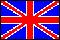ISSN 2079-3537

Scientific Visualization
 Issue Year: 2017 Quarter: 4 Volume: 9 Number: 4 Pages: 13 - 25
 Article Name: BOUNDARY IDENTIFICATION ALGORITHMS AND VISUALIZATION OF THE SOLUTION OF THE PLATEAU PROBLEM IN A BLENDER ENVIRONMENT Authors: E.G. Grigorieva (Russian Federation), A.A. Klyachin (Russian Federation), V.A. Klyachin (Russian Federation) Address: E.G. Grigorieva e_grigoreva@volsu.ru Volgograd State University, Volgograd, Russian Federation A.A. Klyachin klyachin-aa@yandex.ru matf@volsu.ru Volgograd State University, Volgograd, Russian Federation V.A. Klyachin klyachin.va@volsu.ru Volgograd State University, Volgograd, Russian Federation Abstract: In this paper we consider the calculation and construction of the surface having the lowest possible area with the specified edge and the value of the volume of domain that covers this surface. This problem is known from the practice of modern design constructions in which thin shell are often used as coatings or protections. Therefore, one has to solve the problem: How to cover the volume, having a fixed or maximum value, for the minimum area of the its surface. In our work we mathematically formulate this variational problem and present a solution algorithm, using the solutions of the equation of constant mean curvature. The article gives a description of the software implementation of this algorithm, the images calculated surfaces with different computational domain and the boundary conditions. Accuracy of calculations on the average was 0.01%. For the numerical solution of the classical Plateau problem it was built a mathematical model that allows to reduce the problem of minimizing the corresponding functional of the functional area type to the finite-dimensional case. In order to visualize resulting solutions, we consider the possibility of constructing the boundary contours directly in Blender modeling environment. At the same time, the article demonstrates two algorithms for the identification of such circuits for multi-faceted surfaces of an arbitrary topological structure. The implementation of the mentioned algorithms in the simulation environment allows us to calculate of the surface with a minimum value of the functional in interactive mode, and the calculation results are stored in a convenient 3D format. Currently, the built-in module is placed in the public domain on GitHub server and includes the implementation of algorithms for the calculation of minimal surfaces, the capillary surfaces and surfaces of constant mean curvature. Language: English DOI: http://doi.org/10.26583/sv.9.4.02

 Open Article Open Articlein Russian translation Download ZIP archive Download ZIP archivein Russian translation